# Google Sheets IF function - usage and formula examples

The IF function in Google Sheets is one of the easiest functions to learn, and while this holds true, it is also a very helpful one.

In this tutorial, I invite you to take a closer look at how Google Spreadsheet IF function works and what advantages you will get from using it.

## What is the IF function in Google Sheets?

Whenever you use the IF function, you create a decision tree in which certain action follows under one condition, and if that condition is not met – another action follows.

For this purpose, the condition of the function must be in a format of the alternative question with only two possible answers: "yes" and "no".

This is what a decision tree may look like: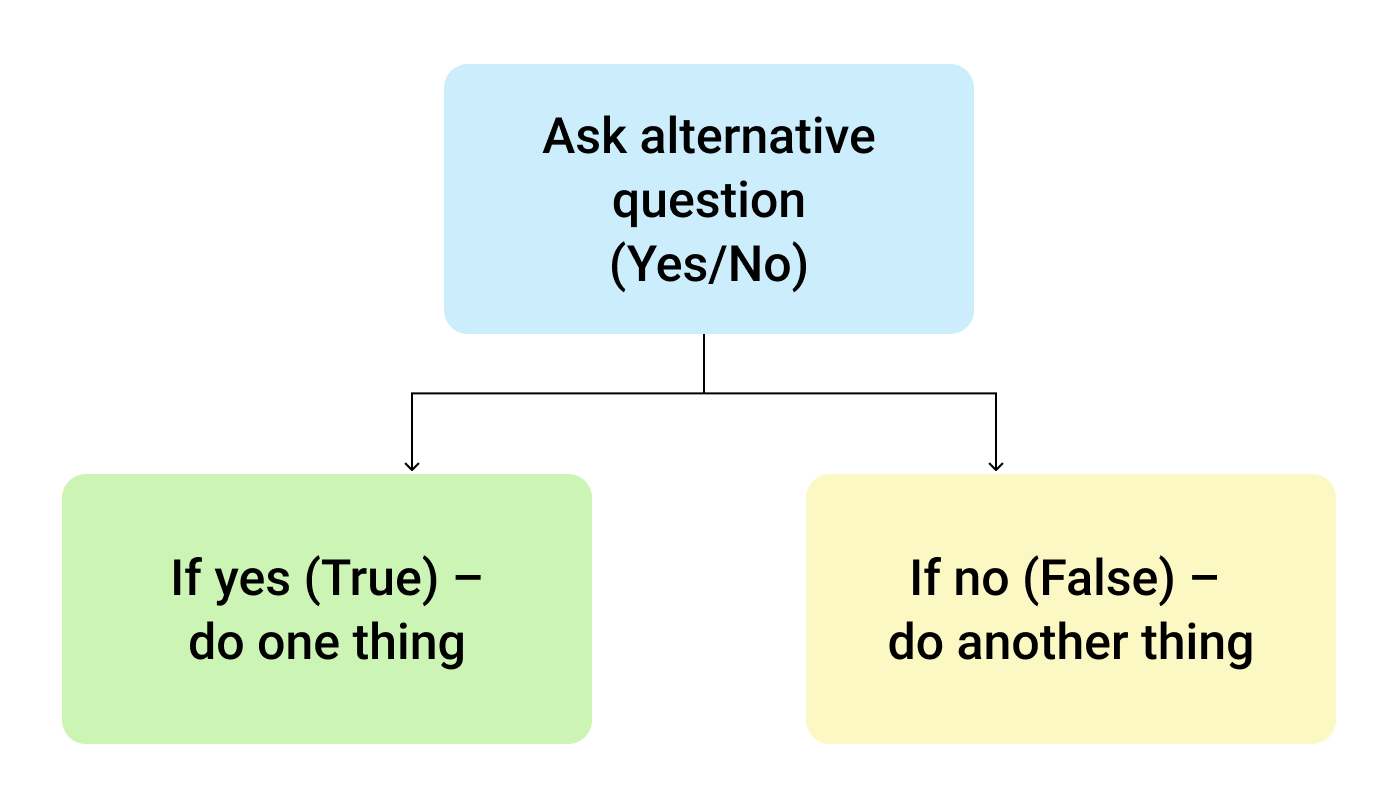So, the IF function allows you to ask a question and indicate two alternative actions depending on the received answer. This question and the alternative actions are known as three arguments of the function.

## IF function syntax in Google Sheets

The syntax for the IF function and its arguments are as follows:

=IF(logical_expression, value_if_true, value_if_false)
• logical_expression – (required) a value or logical expression that is tested to see if it is TRUE or FALSE.
• value_if_true – (required) the operation that is carried out if the test is TRUE.
• value_if_false – (optional) the operation that is carried out if the test is FALSE.

Let's explore the arguments of our IF function in more detail.

The first argument represents a logical question. Google Sheets answers this question with either "yes" or "no", i.e. "true" or "false".

How to formulate the question properly, you may wonder? To do that, you can write a logical expression using such helpful symbols (or comparison operators) as "=", ">", "<", ">=", "<=", "<>". Let us try and ask such a question together.

## Usage of the IF function

Let's assume that you are working in the company selling chocolate in several consumer regions with many clients.

This is what your sales data may look like in Google Sheets: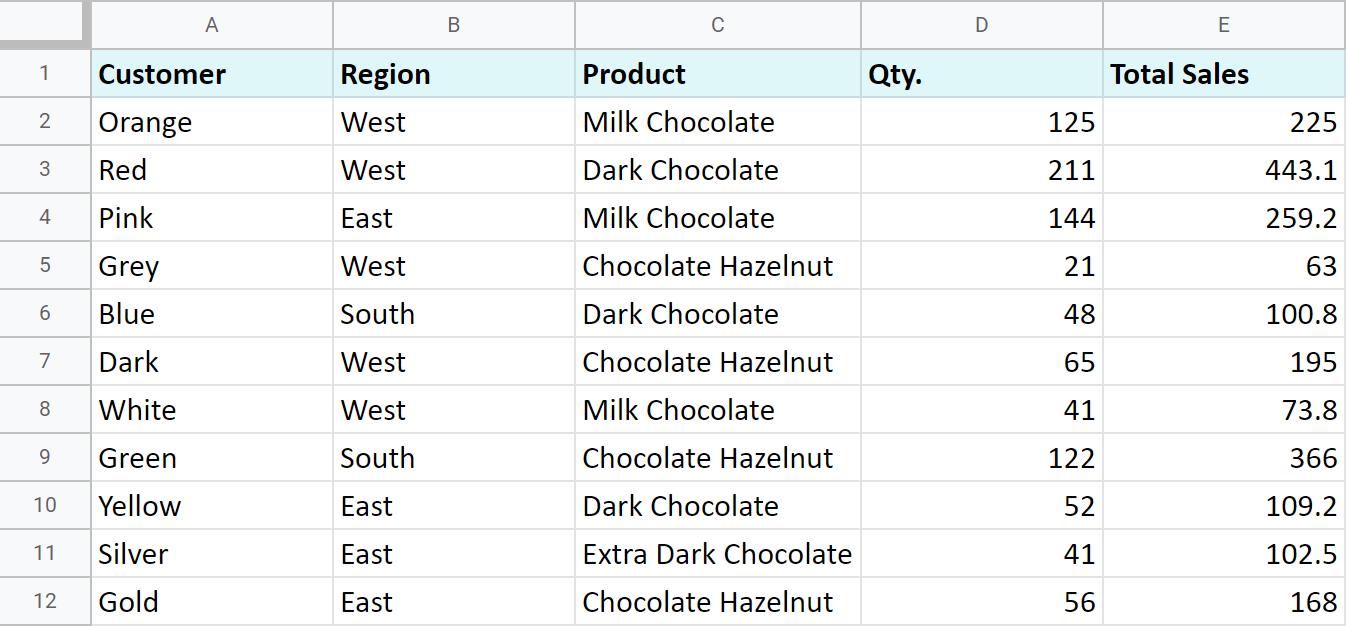Imagine that you need to separate sales made in your local regions from those from abroad. To accomplish that, you should add another descriptive field for each sale – a country where the sales took place. Since there is a lot of data, you need this description field to be created automatically for each entry.

And this is when the IF function comes to play. Let's add the "Country" column to the data table. "West" region represents local sales (Our Country), while the rest are the sales from abroad (Rest of the World).

## How to write out the function properly?

Place the cursor in F2 to make the cell active and type in the equality sign (=). Google Sheets will immediately understand that you are going to enter a formula. That's why right after you type the letter "i" it will prompt you to choose a function that begins with that same letter. And you should choose "IF".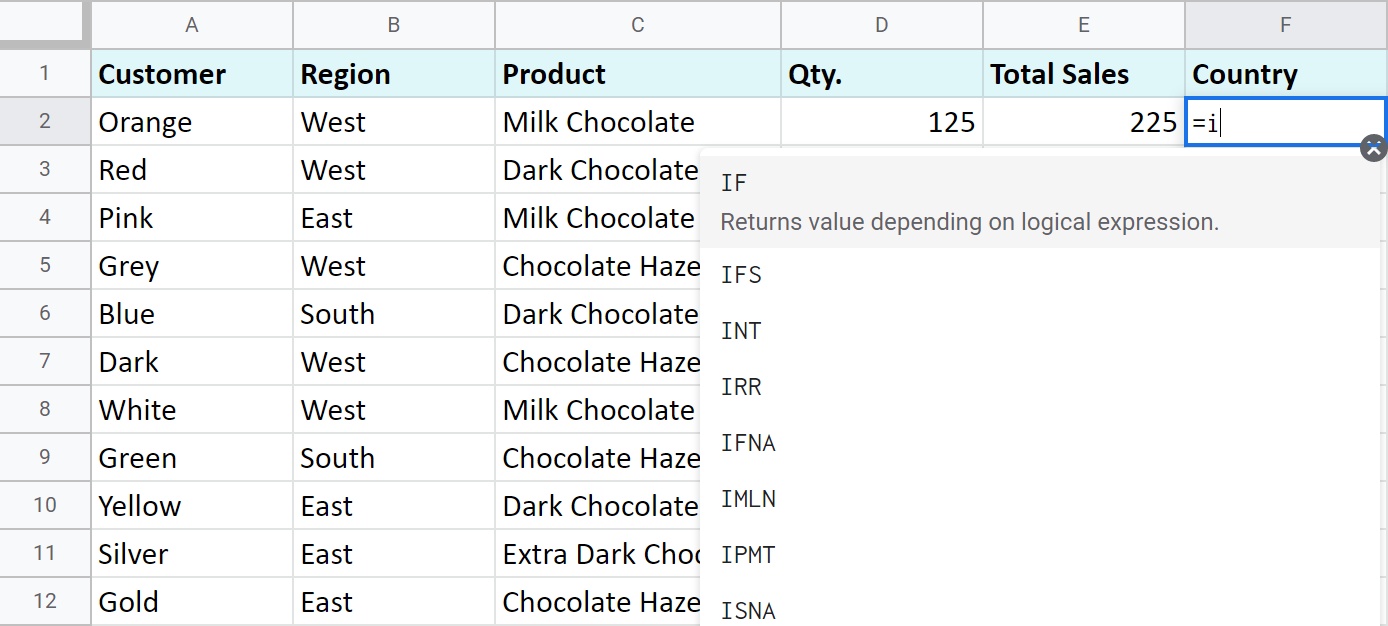After that, all your actions will be accompanied by prompts as well.

For the first argument of the IF function, enter B2="West". As with the other Google Sheets functions, you don't need to enter the address of the cell manually – a mouse click is enough. Then enter comma (,) and specify the second argument.

The second argument is a value that F2 will return if the condition is met. In this case, it will be the text "Our Country".

And again, after the comma, write the value of the 3rd argument. F2 will return this value if the condition is not met: "Rest of the World". Do not forget to finish your formula entry by closing parenthesis ")" and pressing "Enter".

Your entire formula should look like this:

`=IF(B2="West","Our Country","Rest of the World")`

If everything is correct, F2 will return the text "Our Country":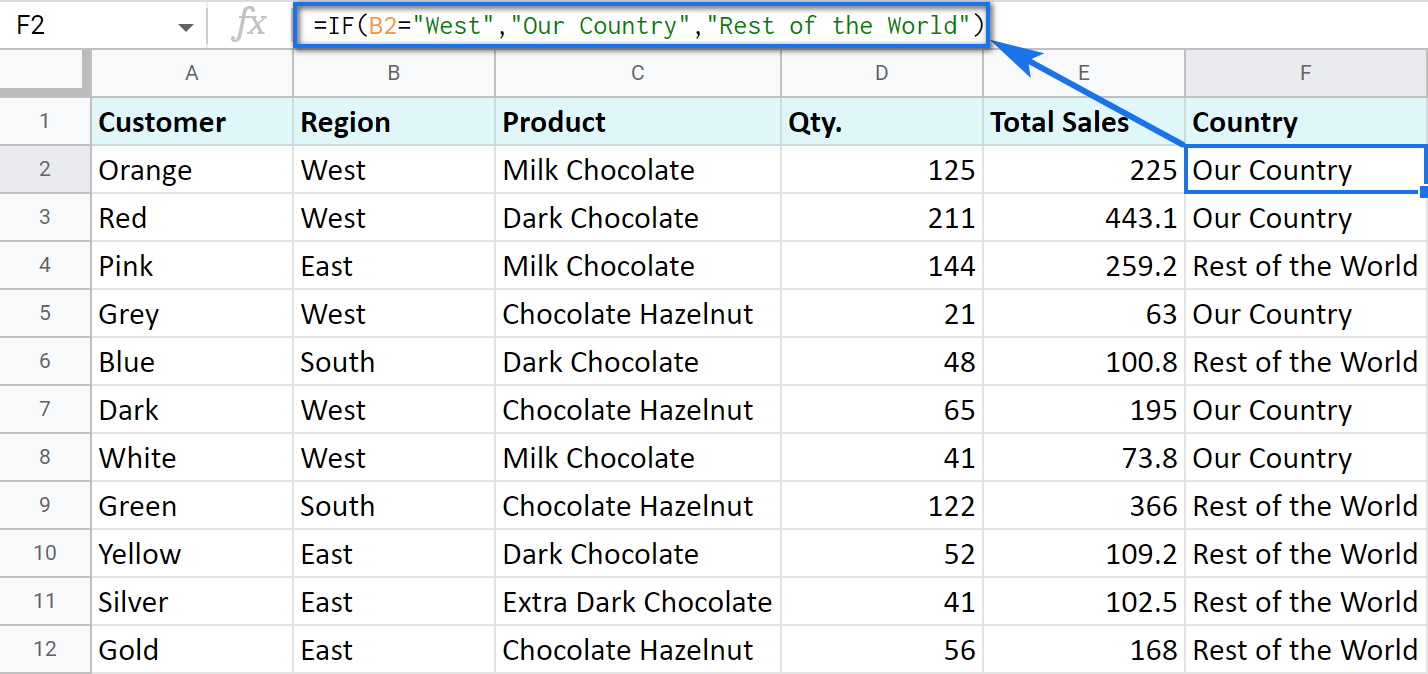Now, all you have to do is to copy this function down column F.

Tip. There's one way to process the entire column with one formula. The ARRAYFORMULA function will help you do that. Using it in the first cell of the column, you can test all cells below against the same condition, and return the corresponding result to each row at the same time:

`=ARRAYFORMULA(IF(B2:B69="West","Our Country","Rest of the World"))`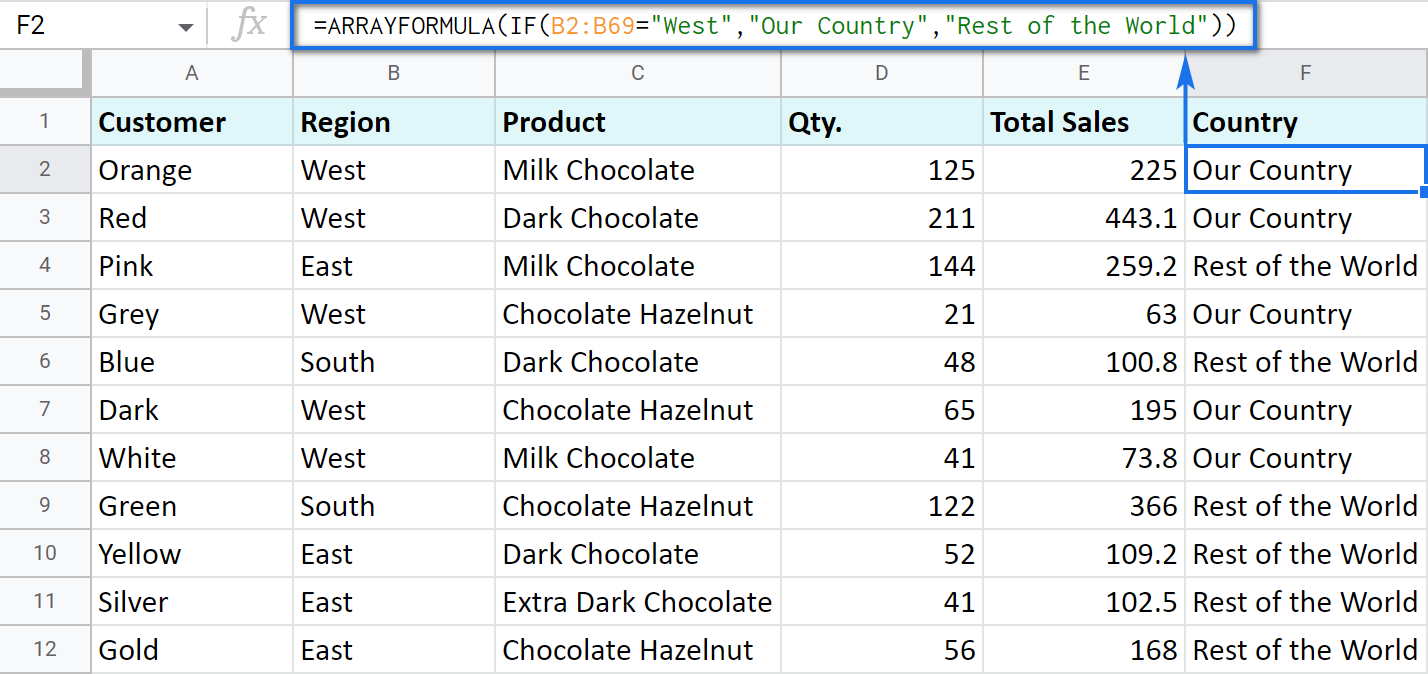Let's examine the other ways of working with the IF function.

## IF function and text values

The usage of the IF function with a text has already been illustrated in the example above.

Note. If the text is being used as the argument, then it must be enclosed in double-quotes.

## IF function and numerical values

You can use numbers for the arguments just as you did with the text.

However, what is very important here is that the IF function makes it possible to not only fill cells with certain numbers based on the conditions met but also calculate.

For example, let's say you offer your clients various discounts based on the total value of the purchase. If the total is more than 200, then the client gets a 10% discount.

For that, you need to use column G and name it "Discount". Then enter the IF function in G2, and the second argument will be represented by the formula that calculates the discount:

`=IF(E2>200,E2*0.1,0)`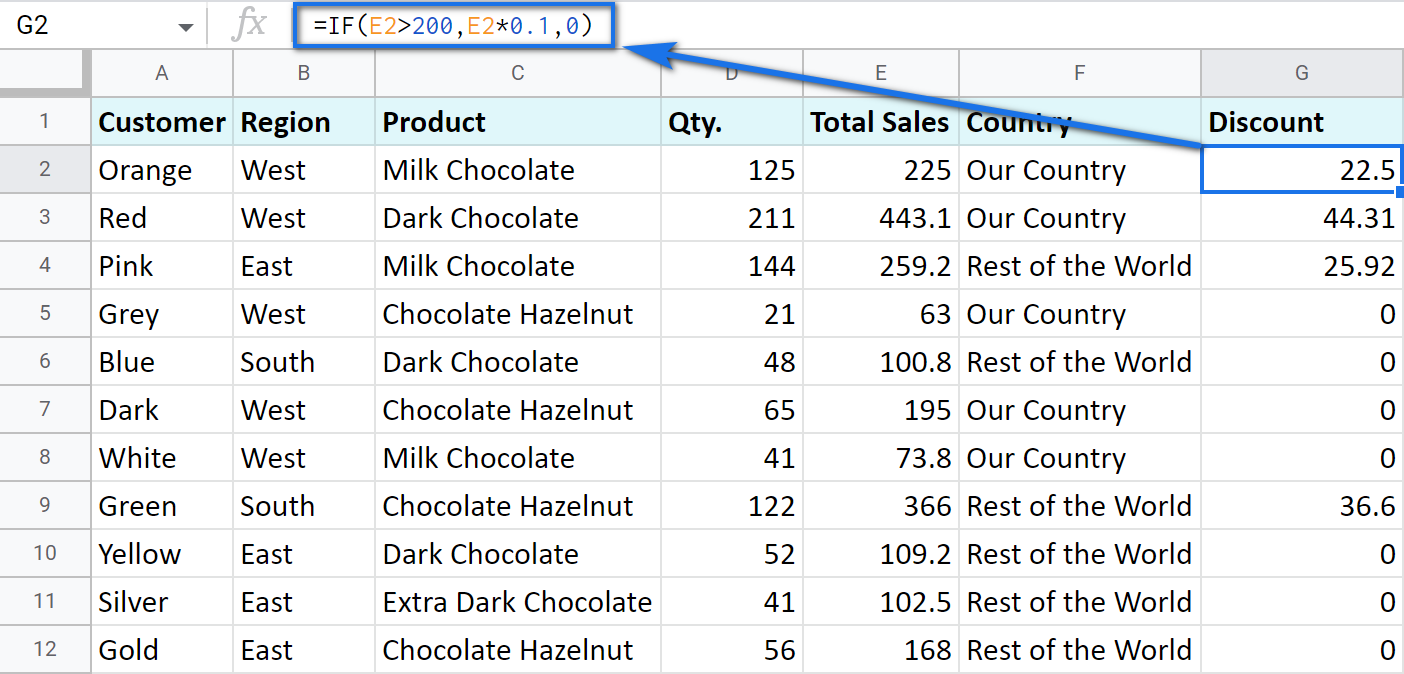## IF blanks/non-blanks

There are cases when your result depends on whether the cell is empty or not. There are two ways to check that:

1. Use the ISBLANK function.

For example, the following formula checks if cells in column E are empty. If so, no discount should be applied, otherwise, it's 5% off:

`=IF(ISBLANK(E2)=TRUE,0,0.05)`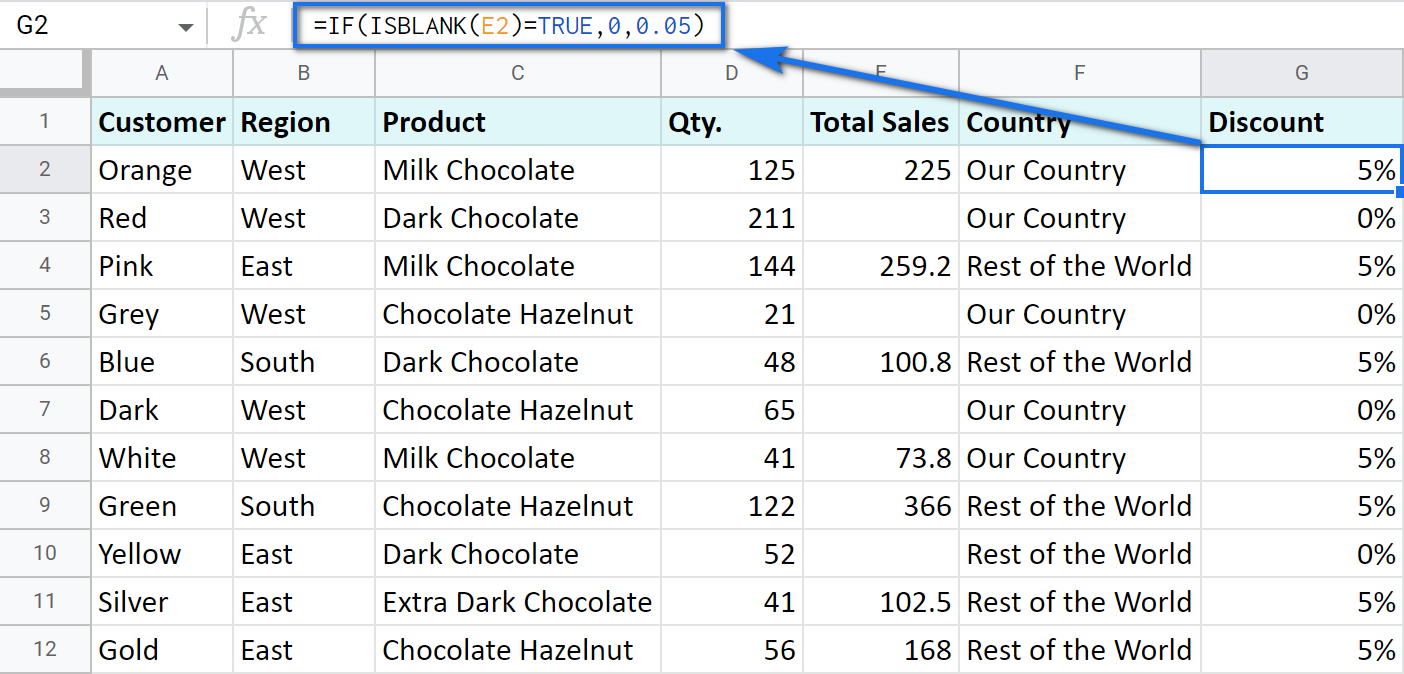Note. If there's a zero-length string in a cell (returned by some formula), the ISBLANK function will result in FALSE.

Here is another formula to check if E2 is empty:

`=IF(ISBLANK(E2)2<>FALSE,0,0.05)`

You can turn the formula the other way around and see if cells are not blank instead:

`=IF(ISBLANK(E2)=FALSE,0.05,0`

`=IF(ISBLANK(E2)<>TRUE,0.05,0)`

2. Use standard comparison operators with a pair of double-quotes:

Note. This method considers zero-length strings (indicated by double-quotes) as empty cells.

`=IF(E2="",0,0.05)` – check if E2 is blank

`=IF(E2<>"",0,0.05)` – check if E2 is not empty.

Tip. In a similar manner, use double-quotes as an argument to return an empty cell by the formula:

`=IF(E2>200,E2*0,"")`

## IF in combination with other functions

As you have already learned, the text, numbers, and formulas can act as the arguments of the IF function. However, other functions can play that role as well. Let's see how it works.

Remember the first way you figured out the country where you sold chocolate? You checked if B2 contained "West".

However, you can build the logic the other way around: list all the possible regions that belong to the "Rest of the World" and check if at least one of them appears in the cell. The OR function in the first argument will help you do that:

=OR(logical_expression1, [logical_expression2, ...])
• logical_expression1 – (required) the first logical value to check for.
• logical_expression2 – (optional) the next logical value to check for.
• and so on.

As you can see, you just enter as many logical expressions as you need to check and the function searches if one of them is true.

To apply this knowledge to the table with sales, mention all the regions that belong to the sales abroad, and the other sales will automatically become local:

`=IF(OR(B2="East",B2="South"),"Rest of the World","Our Country")`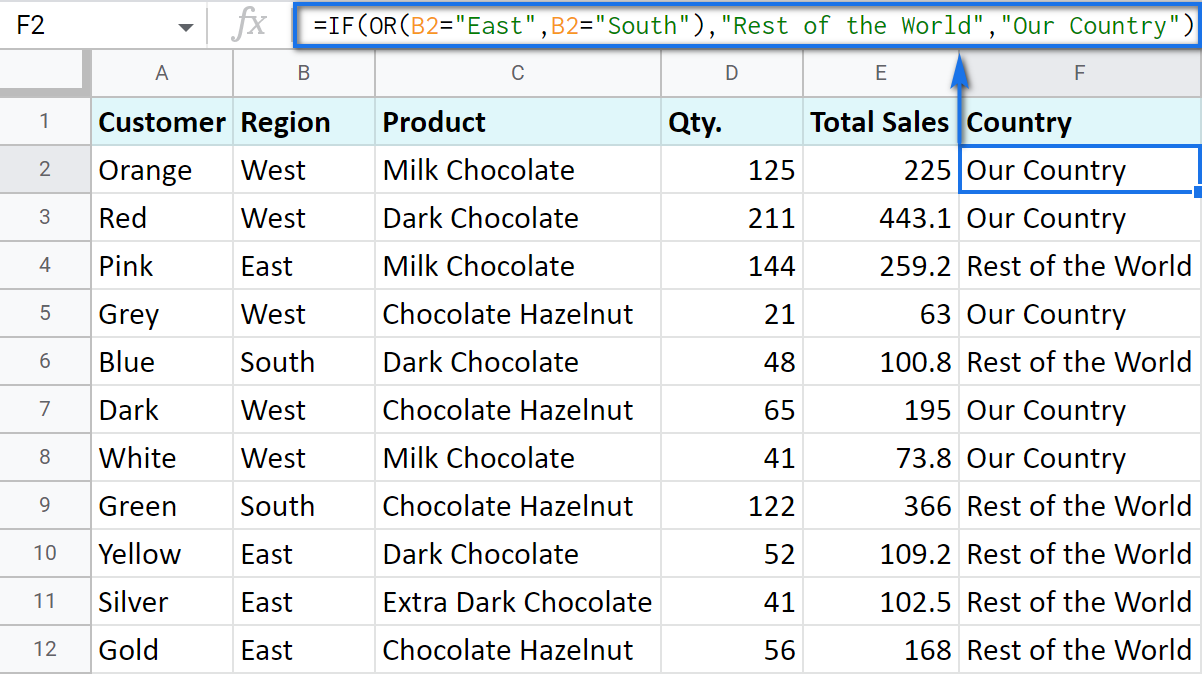The AND function is just as simple. The only difference is that it checks if all listed logical expressions are true:

=AND(logical_expression1, [logical_expression2, ...])

E.g. you need to narrow the search to your town and you know that it is currently buying only hazelnuts. So there are two conditions to consider: region – "West" and product – "Chocolate Hazelnut":

`=IF(AND(B2="West",C2="Chocolate Hazelnut"),"Our Country","Rest of the World")`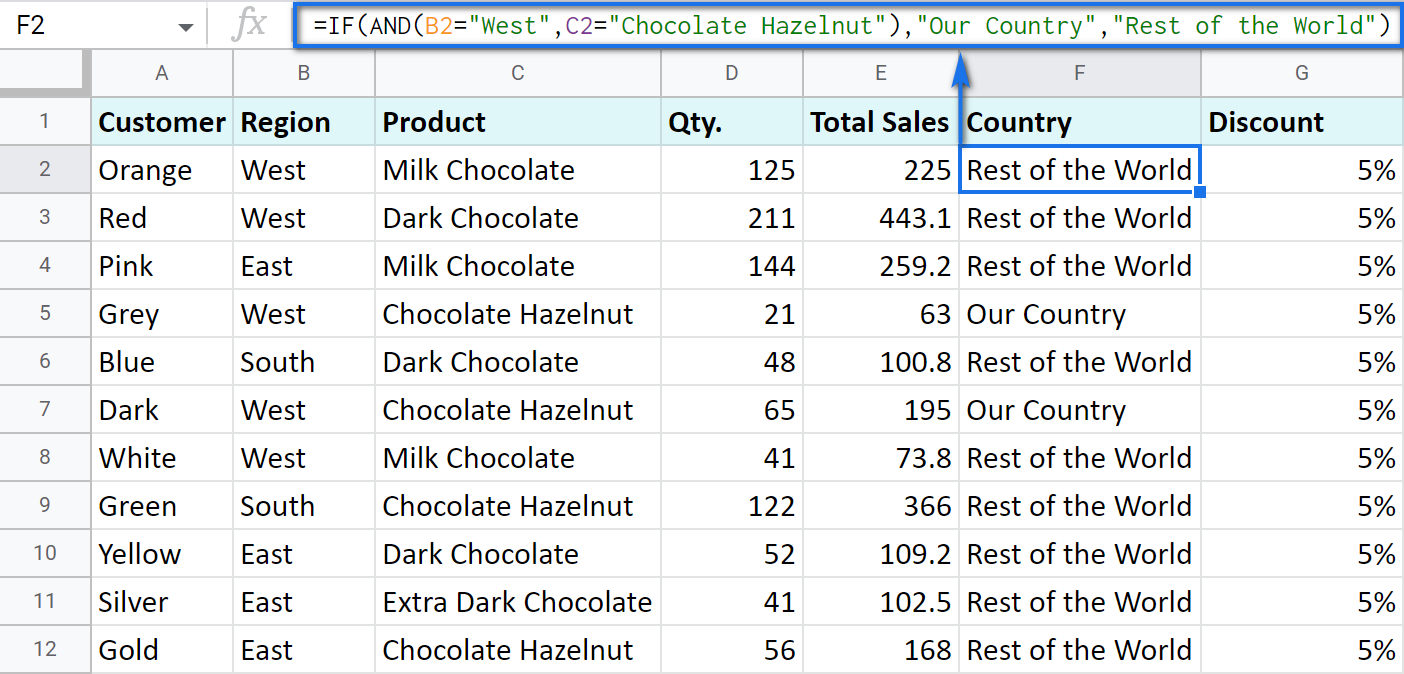### Nested IF formula vs. IFS function for Google Sheets

You can also use the IF function itself as an argument for the bigger IF function.

Let's assume that you have set stricter discount conditions for your clients. If the total purchase is more than 200 units, they get a 10% discount; if the total purchase is between 100 and 199, the discount is 5%. If the total purchase is lower than 100, there is no discount whatsoever.

The following formula shows how the function will look in the cell G2:

`=IF(E2>200,E2*0.1,IF(E2>100,E2*0.05,0))`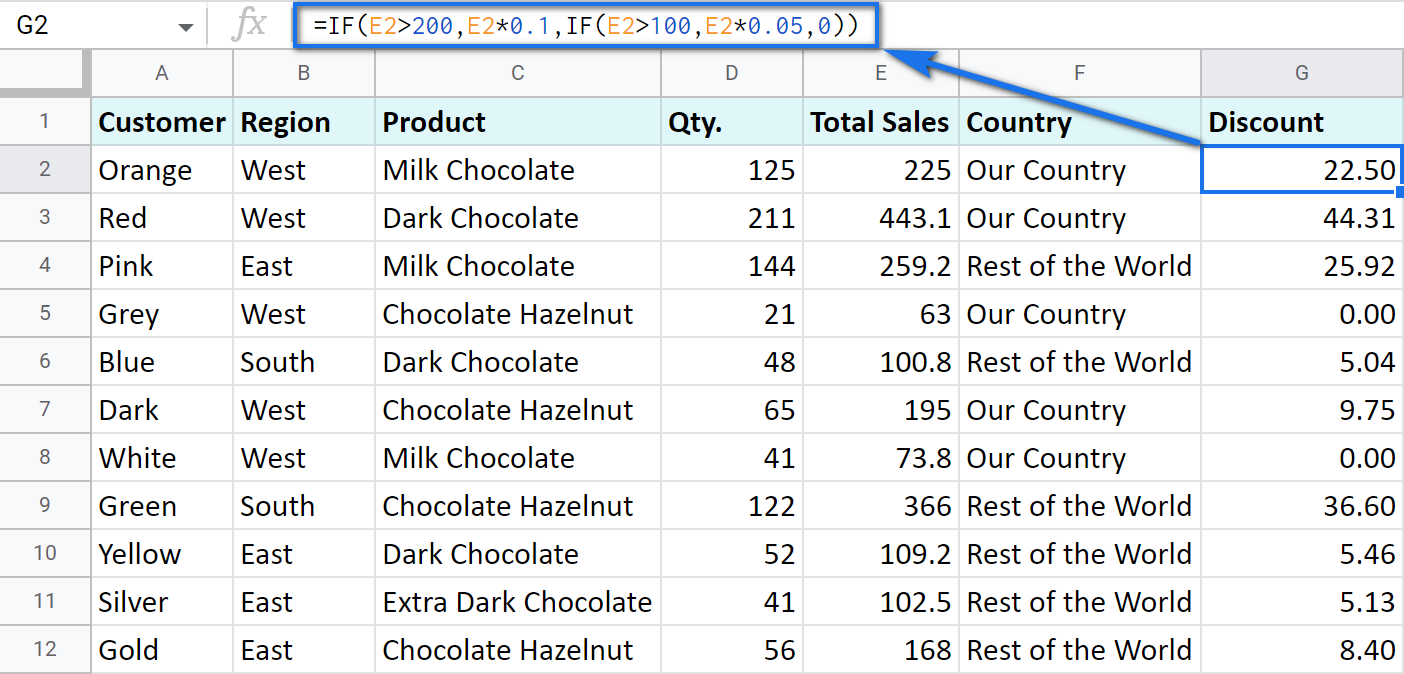Note that it is another IF function that is used as the second argument. In such cases, the decision tree is as follows: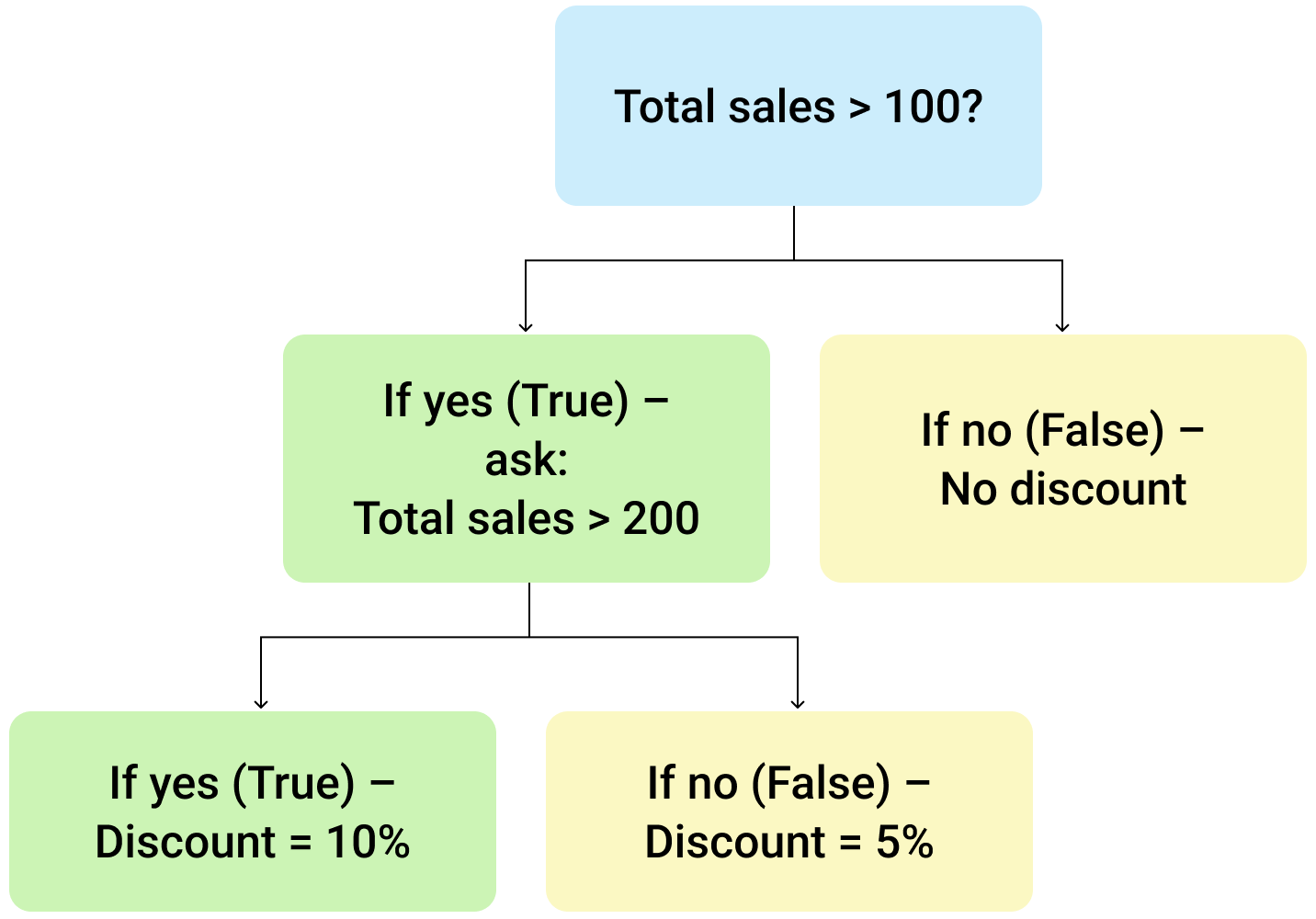Let's make it even more fun and complicate the task. Imagine that you're offering the discounted price to the one region only - "East".

To do that correctly, add the logical expression "AND" to our function. The formula will then look the following way:

`=IF(AND(B2="East",E2>200),E2*0.1,IF(AND(B2="East",E2>100),E2*0.05,0))`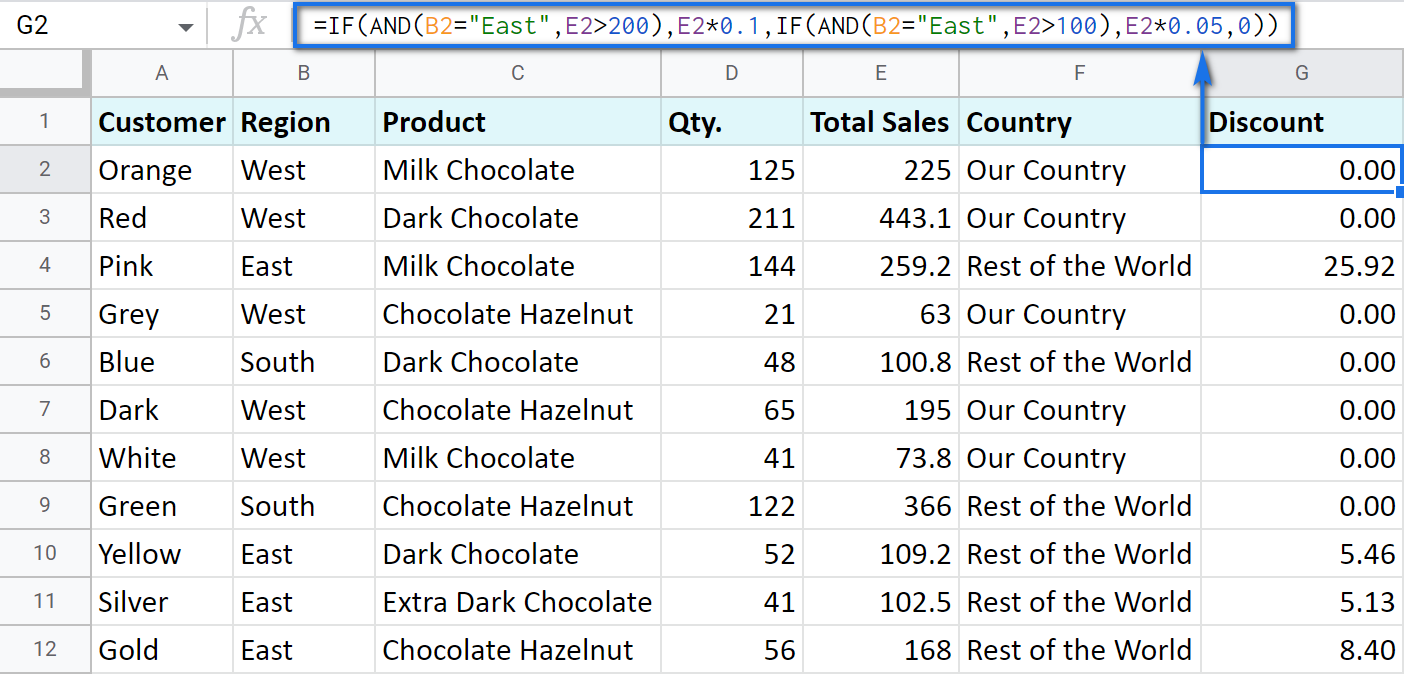As you can see, the number of discounts has reduced greatly while their amount remains intact.

There's also an easier way to write the above thanks to the IFS function:

=IFS(condition1, value1, [condition2, value2, …])
• condition1 – (required) is the logical expression you want to test.
• value1 – (required) is the value to return if the condition1 is true.
• and then you just list conditions with their values to return if they are true.

Here's how the above formula will look with IFS:

`=IFS(AND(B2="East",E2>200),E2*0.1,AND(B2="East",E2>100),E2*0.05)`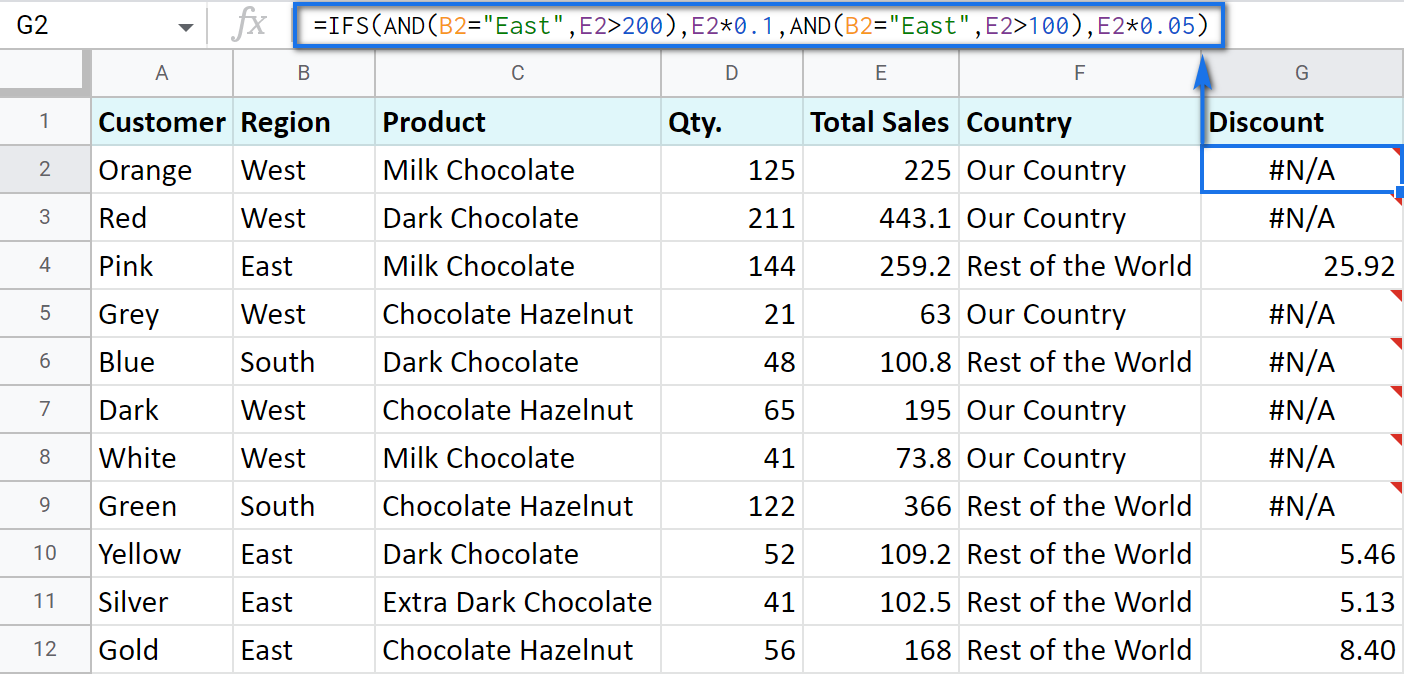Tip. If there's no true condition, the formula will return the #N/A error. To avoid that, wrap your formula with IFERROR:

`=IFERROR(IFS(AND(B2="East",E2>200),E2*0.1,AND(B2="East",E2>100),E2*0.05),0)`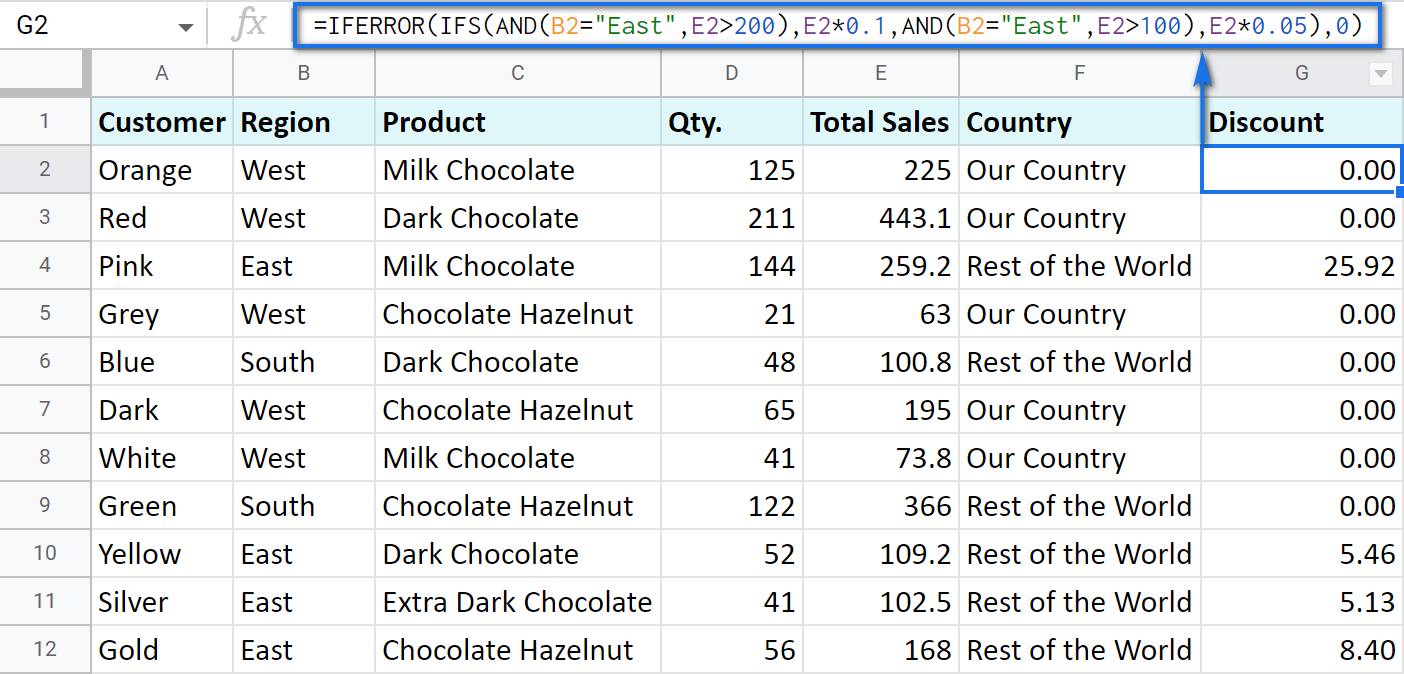#### SWITCH as an alternative to multiple IFs

There's one more function you may want to consider instead of the nested IF: Google Sheets SWITCH.

It checks if your expression corresponds to a list of cases, one by one. When it does, the function returns a corresponding value.

=SWITCH(expression, case1, value1, [case2, value2, ...], [default])
• expression is any cell reference, or a range of cells, or even an actual math expression, or even a text that you'd like to equal to your cases (or test against the criteria). Required.
• case1 is your first criteria to check the expression against. Required.
• value1 is a record to return if the case1 criterion is the same as your expression. Required.
• case2, value2 repeat as many times as criteria you have to check and values to return. Optional.
• default is also completely optional. Use it to see a specific record if none of the cases is met. I'd recommend using it every time to avoid errors when your expression doesn't meet matches among all the cases.

Here are a couple of examples.

To test your cells against a text, use ranges as an expression:

`=ARRAYFORMULA(SWITCH(B2:B69,"West","Our Country","Rest of the World"))`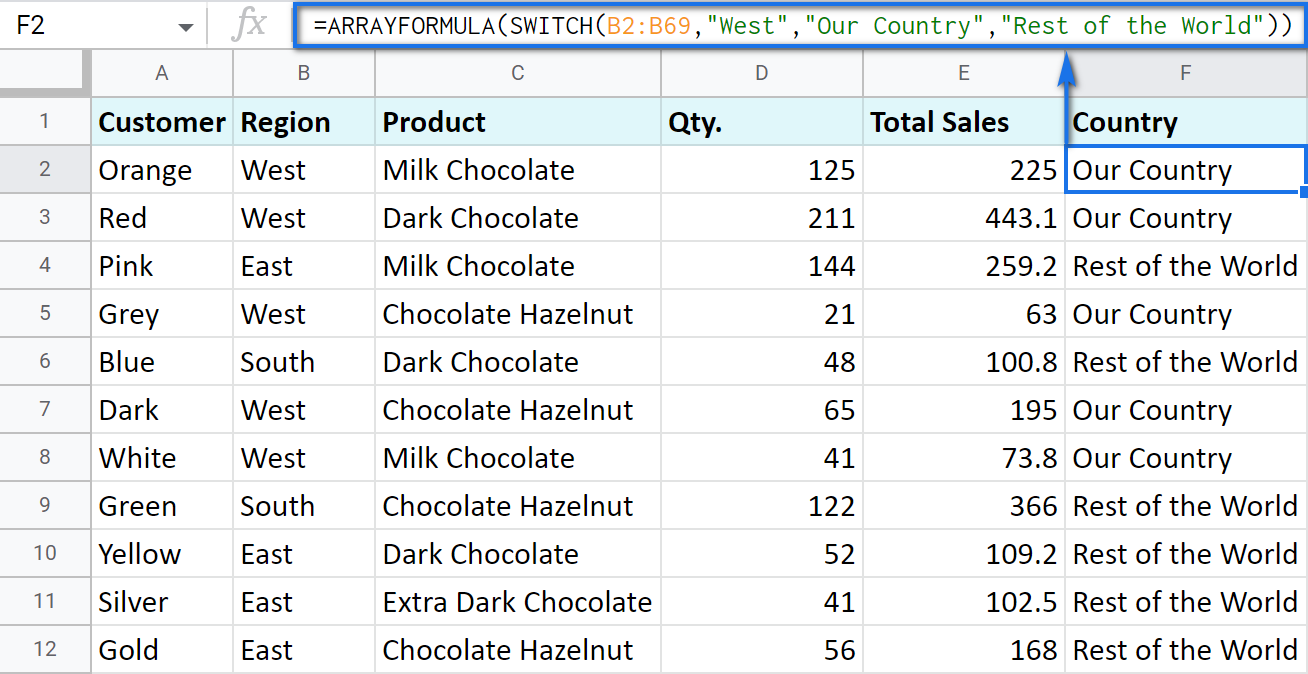In this formula, SWITCH checks what record is in every cell in column B. If it's West, the formula says Our Country, otherwise, Rest of the World. ArrayFormula makes it possible to process the entire column at once.

To work with calculations, it's better to use a boolean expression:

`=SWITCH(TRUE,\$E2>200,\$E2*0.1,AND(\$E2<200,\$E2>100),\$E2*0.05,0)`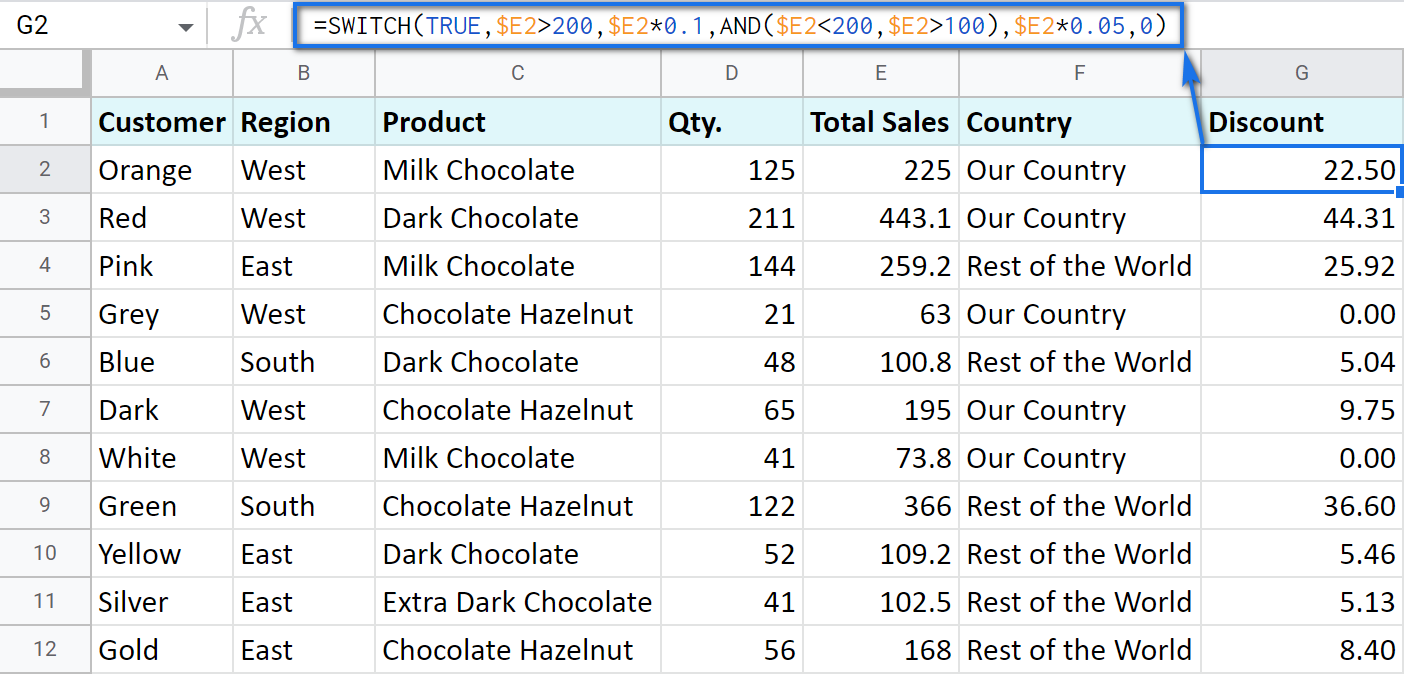Here SWITCH checks if the result of the equation is TRUE or FALSE. When it's TRUE (like if E2 is really greater than 200), I get a corresponding result. If none of the cases in the list is TRUE (meaning they are FALSE), the formula simply returns 0.

Note. SWITCH doesn't know how to calculate the entire range at once, so no ARRAYFORMULA in this case.

### IF statements based on a count

One of the questions we get asked a lot is how to create the IF formula that will return whatever you need if the column contains or doesn't contain a certain record.

For example, check if a customer's name appears more than once in a list (column A) and put the corresponding word (yes/no) into a cell.

A solution is simpler than you may think. You need to introduce the COUNTIF function to your IF:

`=IF(COUNTIF(\$A\$2:\$A\$69,\$A2)>1,"yes","no")`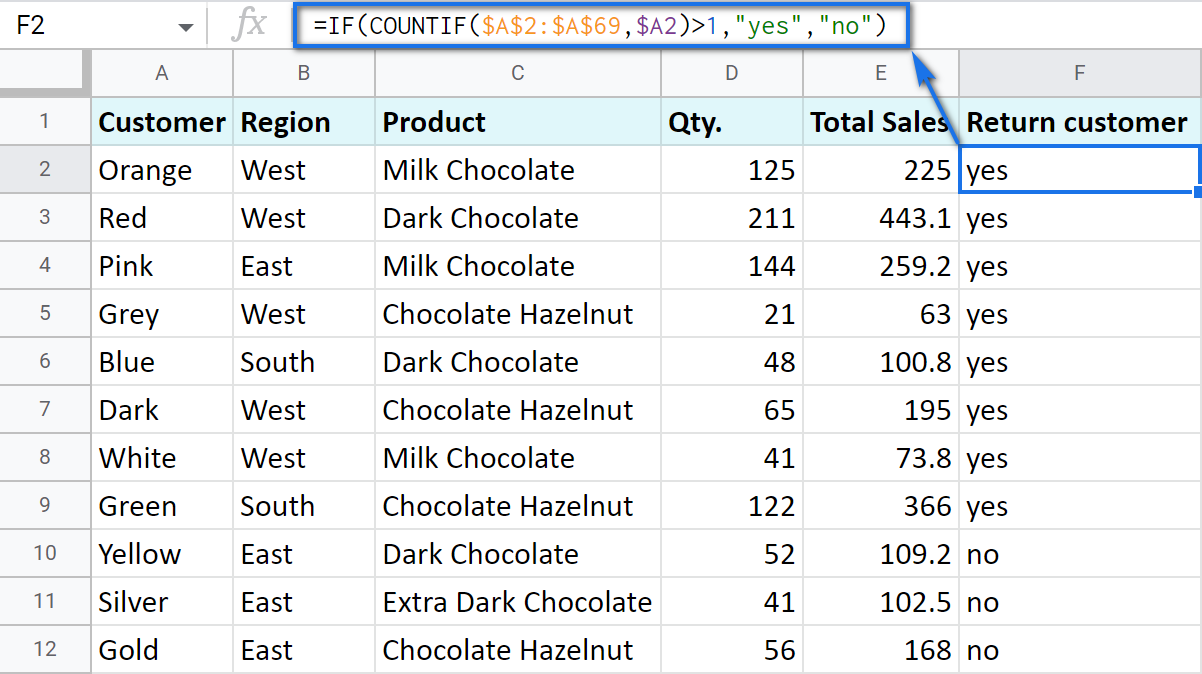## Make Google Sheets build IF formulas for you – IF Formula Builder add-on

If you're tired of keeping track of all those extra characters and proper syntax in formulas, there's another solution available.

IF Formula Builder add-on for Google Sheets offers a visual way of creating IF statements. The tool will handle syntax, extra functions and all required characters for you.

All you need to do is:

• fill blanks with your records one by one. No special treatment for dates, time, etc. Enter them as you always do and the add-on will recognize the data type.
• select required comparison operators from the suggested drop-down lists.
• if needed, add multiple logical expressions in a click: IF OR, IF AND, ELSE IF, THEN IF.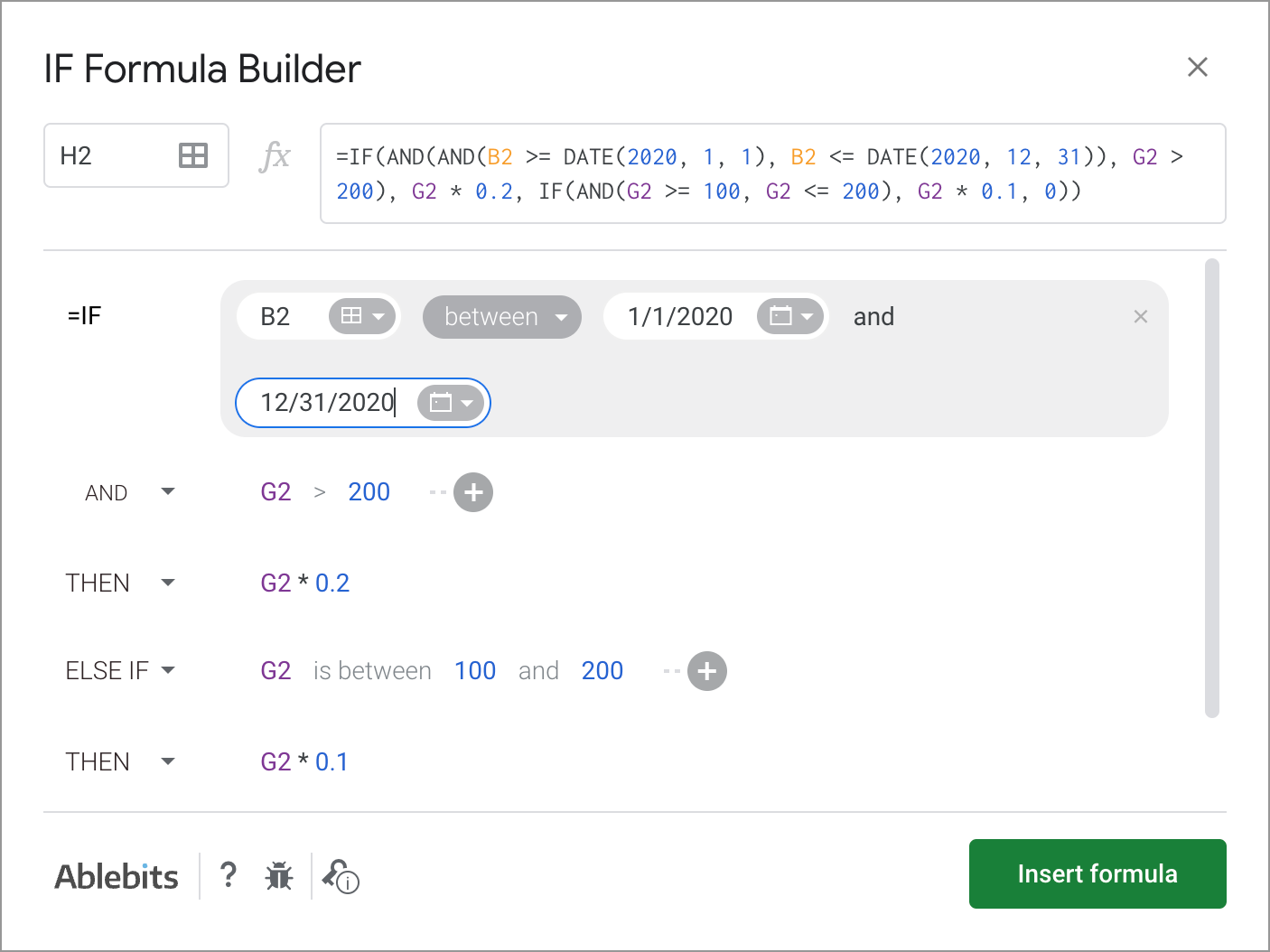As you can see, each logical expression takes its own line. The same goes for true/false outcomes. This reduces the number of possible confusion over the formula drastically.

As you fill everything out, the formula for use will grow in the preview area at the top of the window. To its left, you can select a cell in your sheet where you'd like to have the formula.

When you're ready, paste the formula into the cell of interest by clicking the Insert formula button at the bottom.

Please visit the online tutorial for IF Formula Builder to see all options described in detail.

I hope that there's no room for any doubt now that the IF function, though a very simple one at first glance, opens the door to many options for data processing in Google Sheets. But if you still have questions, feel free to ask them in the comments section down below – we'll be happy to help!

## You may also be interested in

1. Hello, In your first example under the "IF function and numerical values" section. If you wanted cell G2 "Discount", to display "Discount is 22.5". How would you modify the formula =IF(E2>200,E2*0.1,0)? I haven't been able to find how to combine text and a mathematical operation together. I would appreciate your help very much. Thank you in advance.

2. I need formula which used for entry date & timestemp like i fill in A1 Colum then automatically update date in B1 & Time in C1 colum.

• Hello Ravindrag,

I'm sorry but your task is not clear. Please describe it in detail, I'll try to help.

3. Thank you for this post! I'm trying to use multiple if statements with vlookup, but the if statement only runs the first vlookup and then returns an error without evaluating the other two statements:
=if(VLOOKUP(B2, 'SC BC'!\$B:\$B, 1, FALSE)=B2, "SC BC", IF(VLOOKUP(B2, 'SC Prairies'!\$B:\$B, 1, FALSE)=B2, "SC Prairies", IF(VLOOKUP(B2, 'DA Prairies'!\$B:\$B, 1, FALSE)=B2, "DA Prairies", "N/A")

Without the IF statement, the three VLOOKUPs work together. The goal is to identify duplicates and print the location of the duplicate (another tab in the same sheet). Can you help me figure out why the if statements aren't working?

• Hello Emilia,

Note. We keep that Google account for file sharing only and don't monitor its Inbox. Do not email there. Once you share the file, just confirm by replying to this comment.

4. Trying to make a if statement read a total based on individual cells. Example if a1>=6, +1 to d1, if b1>= 4, +1 to d1, if c1>=3, +1 to d1; total is in d1.

• Also with above setting d1=0 to start the loop

• =IF(C4>=18,I4+1, IF(E4>=5,I4+1,IF(G4>=2,I4+1,I4+0)))

Here is the code I have. The problem I am running into is that my total does not reset. For example: if I put in data the code runs and get a total of 2 per say. Then if I change an entry that should give me a total of 1 I get 3. It adds to what is in my total column instead of resetting to 0 first. Also if I start another row the previous row runs another loop and adds to that total again and the new row. Would love to share my doc with you if that helps. Thanks for your help and response.

• Feel free to share an editable copy of your spreadsheet with us (support@apps4gs.com) with a description of your task. I kindly ask you to keep only the list with the formula (remove other lists if there are any) & let me know where the formula is and where the changes should be made that should give a total of 1 but you get 3.

Note. We keep that Google account for file sharing only and don't monitor its Inbox. Do not email there. Once you share the file, just confirm by replying to this comment.

• Doc is shared to your support email. Thanks for your time. Hoping what im asking is possible. Sent details with the share.

• Thank you for sharing the sample, Jamaal. The main problem is that you entered the formulas into I2, I4, I6 but at the same time used these cells in calculations for these formulas. I mean, having =IF(C2>=18,I2+1,IF()) in I2 is incorrect. The formula doesn't know what to take for I2 in order to add 1 while the formula is in I2 itself. So I have to ask you to explain the logic in more detail. What number do you expect to see as a result if C2>=18? Please provide an extra sheet with the actual result you want to see (without formulas, just with numbers, as a reference sheet).

5. Hi, I'm trying to work on our database. And in one column, we have the results of the patients (Positive, Negative, and Awaiting). Whereas, Positive must be confirmed, while Negative and Awaiting must be labeled as suspect. What formula can I use for that. I tried using different if formulas but I think I'm missing out something. By the way, I'm working on google sheet.

• Hi Jessa,

6. How would you get Cell A to show the value of Cell C if Cell B was blank, but Cell B is never blank because it also has a formula in it?

• Hello Kiefer,

It's what the formula in cell B returns that matters, not the fact that there is a formula in cell B.
Try using the second method from this part of the article.